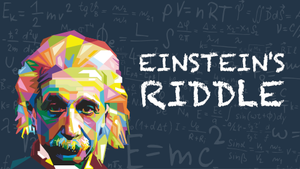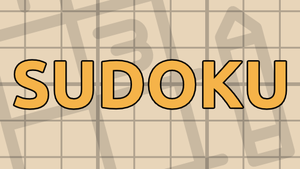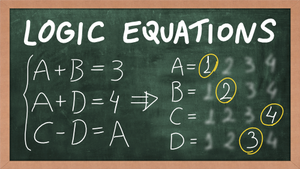1.

The answer that is not an answer to any other question is

• A. A
• B. B
• C. C
• D. D
2.

The only question with the same answer as this one is

• A. 3
• B. 1
• C. 5
• D. 4
3.

The answer to question number 5 is

• A. B
• B. D
• C. A
• D. C
4.

The first question whose answer is A is

• A. 2
• B. 3
• C. 4
• D. 5
5.

The answer to number 3 is

• A. C
• B. B
• C. D
• D. A

#### How to Play

• Click once on an answer to mark it as wrong;
• Click twice to mark it as correct;
• The bar next to each question shows if the question is logically correct (green) or wrong (red).

• Each test has some number of multiple choice questions about itself;
• There is only one correct answer for each question;
• The solution can be found "without too much trial-and-error".

### Need help?

Is it too hard or you don't understand how to play? Try to solve this one:

### More games##### Einstein's Riddle

Einstein said that only 2% of the world could solve this problem. Can you do it?##### Zebra Puzzles

Use logic deductions to solve problems that are similar to the Einstein's Riddle.##### Sudoku

Play the classic japanese logic game.##### Logic Equations

Using logic and basic algebras, solve the equations.